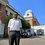# The staircase-covering problemRemove the unit squares lying on a main diagonal of a $(n+1) \times (n+1)$ square grid. You get two congruent shapes, each containing $\frac{n(n+1)}{2}$ unit squares. Call such a shape a staircase of order $n$. (For example, the above image shows a staircase of order $3$)

Let $C(n)$ be the minimum number of rectangles one needs to cover a staircase of order $n$ such that all unit squares in the staircase are covered, no two rectangles overlap, and no part of a rectangle is outside the staircase.

Find an explicit formula for $C(n)$.Note by Shenal Kotuwewatta
6 years, 2 months ago

This discussion board is a place to discuss our Daily Challenges and the math and science related to those challenges. Explanations are more than just a solution — they should explain the steps and thinking strategies that you used to obtain the solution. Comments should further the discussion of math and science.

When posting on Brilliant:

• Use the emojis to react to an explanation, whether you're congratulating a job well done , or just really confused .
• Ask specific questions about the challenge or the steps in somebody's explanation. Well-posed questions can add a lot to the discussion, but posting "I don't understand!" doesn't help anyone.
• Try to contribute something new to the discussion, whether it is an extension, generalization or other idea related to the challenge.

MarkdownAppears as
*italics* or _italics_ italics
**bold** or __bold__ bold
- bulleted- list
• bulleted
• list
1. numbered2. list
1. numbered
2. list
Note: you must add a full line of space before and after lists for them to show up correctly
paragraph 1paragraph 2

paragraph 1

paragraph 2

[example link](https://brilliant.org)example link
> This is a quote
This is a quote
    # I indented these lines
# 4 spaces, and now they show
# up as a code block.

print "hello world"
# I indented these lines
# 4 spaces, and now they show
# up as a code block.

print "hello world"
MathAppears as
Remember to wrap math in $$ ... $$ or $ ... $ to ensure proper formatting.
2 \times 3 $2 \times 3$
2^{34} $2^{34}$
a_{i-1} $a_{i-1}$
\frac{2}{3} $\frac{2}{3}$
\sqrt{2} $\sqrt{2}$
\sum_{i=1}^3 $\sum_{i=1}^3$
\sin \theta $\sin \theta$
\boxed{123} $\boxed{123}$

Sort by:

I think C(n) should be equal to n itself. I guess you could give a straightforward statement based proof where you show separately that it cannot be greater than n, nor can it be less than n.

- 6 years, 2 months ago

We can easily show that $n$ rectangles is sufficient by covering each row with one rectangle. Then, we observe that a staircase of order $n$ has $n$ "stairs", and each of the rectangles can cover at most one stair. So, we need at least $n$ rectangles.

- 6 years, 1 month ago

Precisely what I meant. For some reason, the words 'necessary' and sufficient' eluded me when I made my previous comment :)

- 6 years, 1 month ago

More interesting is the number of ways to cover the staircase with $C(n)$ rectangles (there are $C_n$ ways where $C_n$ is the $n$-th Catalan number).

- 6 years, 2 months ago

I claim that $C(n) = n$.

$C(n) \le n$: We can always tile a staircase with as many rectangles as steps by covering each row with a long rectangle.

$C(n) \ge n$: We can never tile a staircase with less rectangles than steps. Consider some step's top-left corner: this corner must coincide with the top-left corner of a rectangle in the tiling in order for the step to be covered. Thus we must have at least $n$ top-left corners of rectangles in our tiling, or, at least $n$ rectangles.

- 6 years, 1 month ago

Claim: $C(n) = n-1$ (due to the way you indexed it).

There is a one-line proof of that claim. It is left as an exercise to the reader.

Staff - 6 years, 2 months ago

Then wouldn't C(1) = 0? How would we cover a staircase of order 1 with 0 rectangles?

- 6 years, 1 month ago

I don't see why $C(n) = n-1$; a staircase of order $n$ has $n$ rows.

- 6 years, 2 months ago

I didn't understand the "divided by one of it's main diagonals". Won't it be more of a $n \times (n+1)$ rectangle instead?

Though, given that he says the image has order 3, I guess he did pick the natural ordering.

Staff - 6 years, 2 months ago

I take it as "cut the square with one diagonal, and remove the squares that are also cut apart with the diagonal; the rest is two congruent pieces, each piece is a staircase".

- 6 years, 1 month ago

That is what I meant.

- 6 years, 1 month ago

jee advance2015 paper 2 ellipse question

- 6 years, 1 month ago